# Class 10 RD Sharma Solutions – Chapter 2 Polynomials – Exercise 2.3

• Last Updated : 20 May, 2021

### Question 1. Apply division algorithm to find the quotient q(x) and remainder r(x) on dividing f(x) by g(x) in each of the following:

(i) f(x) = x3 – 6x2 + 11x – 6, g(x) = x2 + x + 1

(ii) f(x) = 10x4 + 17x3 – 62x2 + 30x – 105, g(x) = 2x2 + 7x + 1

Attention reader! All those who say programming isn't for kids, just haven't met the right mentors yet. Join the  Demo Class for First Step to Coding Coursespecifically designed for students of class 8 to 12.

The students will get to learn more about the world of programming in these free classes which will definitely help them in making a wise career choice in the future.

(iii) f(x) = 4x3 + 8x2 + 8x + 7, g(x) = 2x2 – x + 1

(iv) f(x) = 15x3 – 20x2 + 13x – 12, g(x) = x2 – 2x + 2

Solution:

(i) Here we have to divide f(x) = x3 – 6x2 + 11x – 6 by g(x) = x2 + x + 1

So, to get quotient q(x) and remainder r(x), we use division algorithm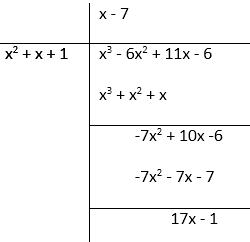Therefore,

Remainder r(x) = 17x – 1

Quotient q(x) = x – 7

(ii) Here we have to divide f(x) = 10x4 + 17x3 – 62x2 + 30x – 105 by g(x) = 2x2 + 7x + 1

So, to get quotient q(x) and remainder r(x), we use division algorithm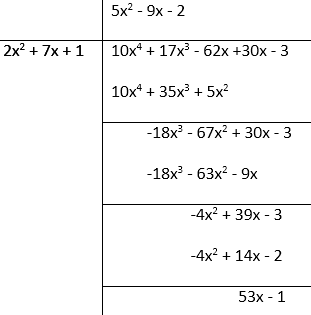Therefore,

Remainder r(x) = 53x – 1

Quotient q(x) = 5x2 – 9x – 2

(iii) Here we have to divide f(x) = 4x3 + 8x2 + 8x + 7 by g(x) =2x2 – x + 1

So, to get quotient q(x) and remainder r(x), we use division algorithm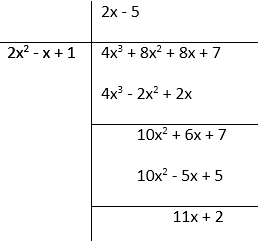Therefore,

Remainder r(x) = 11x + 2

Quotient q(x) = 2x – 5

(iv) f(x) = 15x3 – 20x2 + 13x – 12, g(x) = x2 – 2x + 2

Here we have to divide f(x) =  15x3 – 20x2 + 13x – 12 by g(x) = x2 – 2x + 2

So, to get quotient q(x) and remainder r(x), we use division algorithm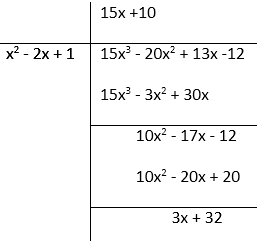Therefore,

Remainder r(x) = 3x + 32

Quotient q(x) = 15x + 10

### Question 2. Check whether the first polynomial is a factor of the second polynomial by applying the division algorithm:

(i) g(t) = t2 – 3; f(t) = 2t4 + 3t3 – 2t2 – 9t – 12

(ii) g(x) = x2 – 3x + 1; f(x) = x5 – 4x3 + x2 + 3x + 1

(iii) g(x) = 2x2 – x + 3; f(x) = 6x5 − x4 + 4x3 – 5x2 – x – 15

Solution:

(i) Here, we have to check whether g(t) = t2 – 3 is a factor of f(t) = 2t4 + 3t3 – 2t2 – 9t – 12

So, by using division algorithm, we get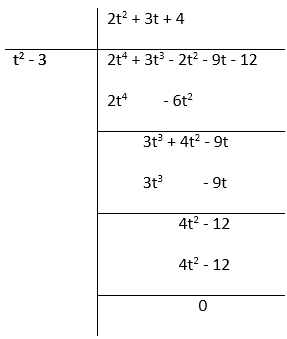As the remainder left is 0.

Therefore,

g(t) = t2 – 3 is a factor of f(t) = 2t4 + 3t3 – 2t2 – 9t – 12

(ii) Here, we have to check whether g(x) = x2 – 3x + 1 is a factor off(x) = x5 – 4x3 + x2 + 3x + 1

So, by using division algorithm, we get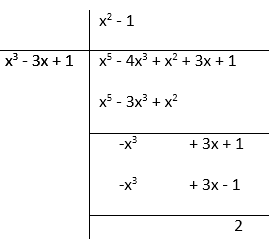As the remainder left is 2.

Therefore,

g(x) = x2 – 3x + 1 is not a factor of  f(x) = x5 – 4x3 + x2 + 3x + 1

(iii) Here, we have to check whether g(x) = 2x2 – x + 3 is a factor of f(x) = 6x5 − x4 + 4x3 – 5x2 – x – 15

So, by using division algorithm, we get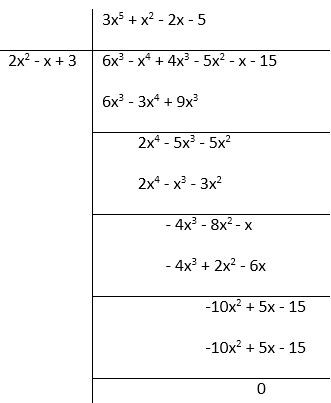As the remainder left is 0.

Therefore,

g(x) = 2x2 – x + 3 is a factor of f(x) = 6x5 − x4 + 4x3 – 5x2 – x – 15

### Question 3. Obtain all zeroes of the polynomial f(x) = f(x) = 2x4 + x3 – 14x2 – 19x – 6, if two of its zeroes are -2 and -1.

Solution:

Given: f(x) = 2x4 + x3 – 14x2 – 19x – 6

Here we have given the two zeroes of the polynomial that are -2 and -1,

Hence, its factors will be  (x + 2) and (x + 1)

Further,

(x + 2)(x + 1) = x2 + x + 2x + 2 = x2 + 3x + 2

So, by using division algorithm, we get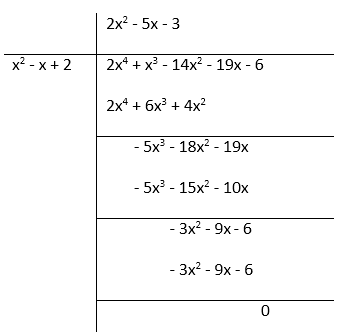f(x) = 2x4 + x3 – 14x2 – 19x – 6 = (2x2 – 5x – 3)(x2 + 3x + 2)

= (2x + 1)(x – 3)(x + 2)(x + 1)

Hence, the factors of f(x) = 2x4 + x3 – 14x2 – 19x – 6 are (2x + 1), (x – 3), (x + 2), (x + 1)

Therefore, the zeroes of the polynomial are -1/2, 3, -2, -1

### Question 4. Obtain all zeroes of f(x) = x3 + 13x2 + 32x + 20, if one of its zeroes is -2.

Solution:

We have been given the zero of the polynomial f(x) = x3 + 13x2 + 32x + 20 is -2.

Hence, its factor is (x + 2).

So, by using division algorithm, we get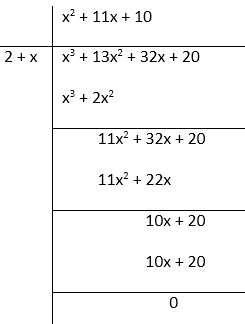Thus,

f(x) = x3 + 13x2 + 32x + 20

= (x2 + 11x + 10)(x + 2)

= (x2 + 10x + x + 10)(x + 2)

= (x + 10)(x + 1)(x + 2)

Hence, the factors of f(x) = x3 + 13x2 + 32x + 20 are (x + 10), (x + 1), (x + 2)

Thus, the zeroes of the polynomial are -1, -10, -2.

### Question 5. Obtain all zeroes of the polynomial f(x) = x4 – 3x3 – x2 + 9x – 6, if the two of its zeroes are -√3 and √3.

Solution:

Here, we are given two zeros of the polynomial f(x) = x4 – 3x3 – x2 + 9x – 6 that are -√3 and √3.

Thus, the factors are (x + √3​)(x − √3​) ⇒ x2 – 3.

So, by using division algorithm, we get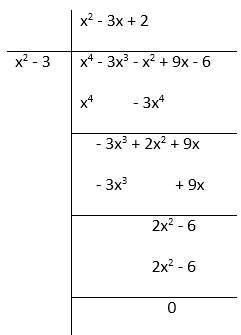Hence,

f(x) = x4 – 3x2 – x2 + 9x – 6 = (x2 – 3)(x2 – 3x + 2)

(x + √3)(x – √3)(x2 – 2x – 2 + 2)

= (x + √3)(x – √3)(x – 1)(x – 2)

Thus, the factors of f(x) = x4 – 3x3 – x2 + 9x – 6 are (x + √3)(x – √3)(x – 1)(x – 2).

Therefore, the zeroes of the polynomial are -√3, √3, 1, 2.

### Question 6. Obtain all zeroes of the polynomial f(x) = 2x4 – 2x3 – 7x2 + x – 1, if the two of its zeroes are -√3/2 and √3/2.

Solution:

Here, we are given two zeros of the polynomial f(x) = 2x4 – 2x3 – 7x2 + x – 1 that are -√3/2 and √3/2.

Thus, the factors are⇒ x2 – 3/2.

So, by using division algorithm, we get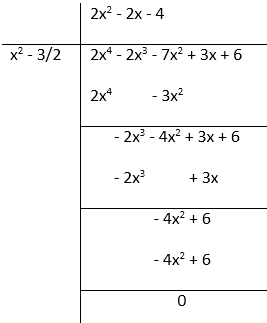Hence,Factors of f(x) = 2x4 – 2x3 – 7x2 + x – 1 are.

Thus, the zeroes of the polynomial are -1, 2, -√3/2 and √3/2.

### Question 7. Find all the zeroes of the polynomial x4 + x3 – 34x2 – 4x + 120, if the two of its zeroes are 2 and – 2.

Solution:

Here, we are given two zeros of the polynomial x4 + x3 – 34x2 – 4x + 120 that are 2 and -2.

Thus, the factors are (x + 2)(x – 2)⇒ x2 – 4.

So, by using division algorithm, we get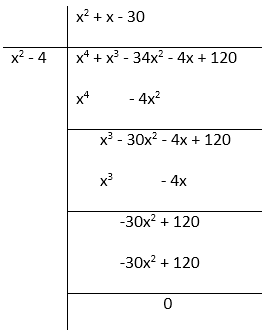Hence,

x4 + x3 – 34x2 – 4x + 120 = (x2 – 4)(x2 + x – 30)

= (x – 2)(x + 2)(x2 + 6x – 5x – 30)

=  (x – 2)(x + 2)(x + 6)(x – 5)

So, the factors of x4 + x3 – 34x2 – 4x + 120 are (x – 2), (x + 2), (x + 6), (x – 5)

Thus, the zeroes of the polynomial = x = 2, – 2, – 6, 5

### Question 8. Find all the zeroes of the polynomial 2x4 + 7x3 – 19x2 – 14x + 30, if the two of its zeroes are √2 and -√2.

Solution:

Here, we are given two zeros of the polynomial 2x4 + 7x3 – 19x2 – 14x + 302 that are √2 and -√2.

Thus, the factors are (x + √2)(x – √2) ⇒ x2 – 2.

So, by using division algorithm, we get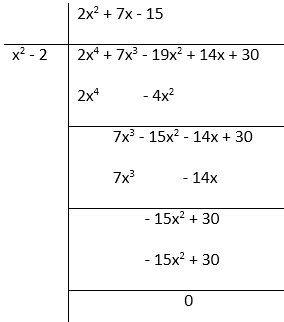Hence,

2x4 + 7x3 – 19x2 – 14x + 30 = (x2 – 2)(2x2 + 7x – 15)

= (2x2 + 10x – 3x – 15)(x + √2)(x – √2)

= (2x – 3)(x + 5)(x + √2)(x – √2)

So, the factors of 2x4 + 7x3 – 19x2 – 14x + 30 are (2x – 3), (x + 5), (x + √2), (x – √2)

Thus, the zeroes of the polynomial is √2, -√2, -5, 3/2.

### Question 9. Find all the zeroes of the polynomial f(x) = 2x3 + x2 – 6x – 3, if two of its zeroes are -√3 and √3.

Solution:

Here, we are given two zeros of the polynomial f(x) = 2x3 + x2 – 6x – 3 that are -√3 and √3.

Thus, the factors are (x + √3)(x – √3) ⇒ x2 – 3.

So, by using division algorithm, we get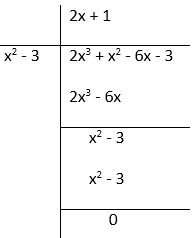Hence,

f(x) = 2x3 + x2 – 6x – 3

= (x2 – 3)(2x + 1)

= (x + √3)(x – √3)(2x + 1)

Factors of f(x) = 2x3 + x2 – 6x – 3 are (x + √3), (x – √3), 2x + 1

Thus, the zeroes for the given polynomial are √3, -√3, -1/2

### Question 10. Find all the zeroes of the polynomial f(x) = x3 + 3x2 – 2x – 6, if the two of its zeroes are √2 and -√2.

Solution:

Here, we are given two zeros of the polynomial f(x) = x3 + 3x2 – 2x – 6 that are √2 and -√2.

Thus, the factors are (x + √2)(x – √2)⇒ x2 – 2.

So, by using division algorithm, we get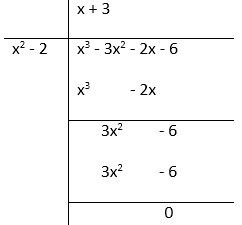Hence,

f(x) = x3 + 3x2 – 2x – 6

= (x2 – 2)(x + 3)

= (x + √2)(x – √2)(x + 3)

Factors of f(x) = x3 + 3x2 – 2x – 6 are (x + √2), (x – √2), (x + 3)

Thus, the zeroes of the given polynomial is -√2, √2, and – 3.

### Question 11. What must be added to the polynomial f(x) = x4 + 2x3 – 2x2 + x − 1 so that the resulting polynomial is exactly divisible by g(x) = x2 + 2x − 3.

Solution:

Here we have to add to the polynomial f(x) = x4 + 2x3 – 2x2 + x − 1 so that the

resulting polynomial is exactly divisible by g(x) = x2 + 2x − 3.

So, divide f(x) = x4 + 2x3 – 2x2 + x − 1 by g(x) = x2 + 2x − 3 to get the answer.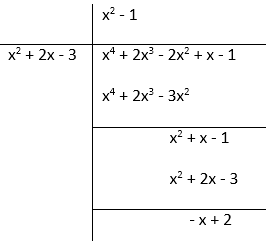As the remainder left is (x – 2) to get the resulting polynomial exactly divisible by

g(x) = x2 + 2x − 3 we must add (x – 2) to f(x) = x4 + 2x3 – 2x2 + x − 1.

### Question 12. What must be subtracted from the polynomial f(x) = x4 + 2x3 – 13x2 –12x + 21 so that the resulting polynomial is exactly divisible by g(x) = x2 – 4x + 3.

Solution:

Here we have to subtract to the polynomial f(x) = x4 + 2x3 – 13x2 – 12x + 21

so that the resulting polynomial is exactly divisible by g(x) = x2 – 4x + 3.

So, divide f(x) = x4 + 2x3 – 13x2 – 12x + 21 by  g(x) = x2 – 4x + 3 to get the answer.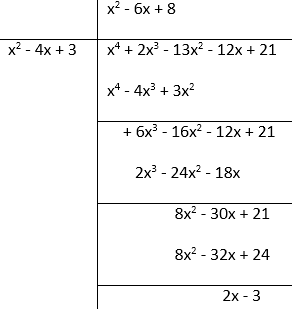As the remainder left is (2x – 3) to get the resulting polynomial exactly divisible by

g(x) = x2 – 4x + 3 we must add (2x – 3) to f(x) = x4 + 2x3 – 13x2 – 12x + 21.

### Question 13. Given that √2 is a zero of the cubic polynomial f(x) = 6x3 + √2x2– 10x – 4√2, find its other two zeroes.

Solution:

Here, we are given that √2 is the zero of the cubic polynomial

f(x) = 6x3 + √2x2– 10x – 4√2, thus, factor of the polynomial is (x – √2)

So, by using division algorithm, we get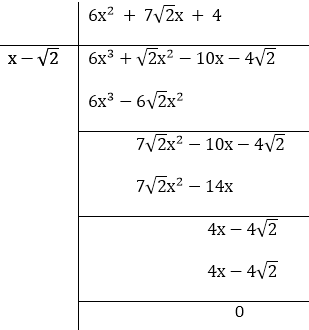Hence,

f(x) = 6x3 + √2x2 – 10x – 4√2

= (x – √2)(6x2 + 7√2x + 4)

= (x – √2)(6x2 + 4√2x + 3√2x + 4)

= (x – √2)(3x + 2√2)(2x + √2)

The factors of f(x) = 6x3 + √2x2– 10x – 4√2 are (x – √2), (3x + 2√2), (2x + √2)

Therefore, the zeros of the polynomial are -2√2/3, -√2/2, √2

### Question 14. Given that x – √5 is a factor of the cubic polynomial x3 – 3√5x2 + 13x – 3√5, find all the zeroes of the polynomial.

Solution:

Here, we have x – √5 as factor of the cubic polynomial x3 – 3√5x2 + 13x – 3√5

To find all the zeros of the polynomial, we have to divide the polynomial x3 – 3√5x2 + 13x – 3√5 by the factor x – √5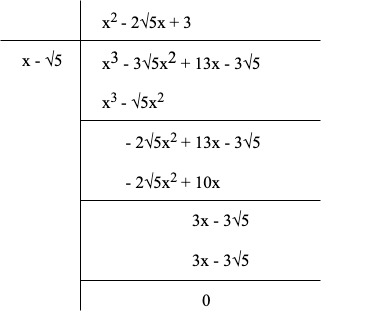Hence,

x3 – 3√5x2 + 13x – 3√5

= (x – √5)(x2 – 2√5 + 3)

= (x – √5)(x – (√5 + √2))(x – (√5 – √2))

So, the factors of the cubic polynomial x3 – 3√5x2 + 13x – 3√5 are (x – √5), (x – (√5 + √2)), (x – (√5 – √2))

Therefore, the zero of the polynomial are √5, (√5 – √2), (√5 + √2)

My Personal Notes arrow_drop_up